## GATE 2015 [Set-3]

 Question 1

Extreme focus on syllabus and studying for tests has become such a dominant concern of Indian students that they close their minds to anything _____ to the requirements of the exam

 A related B extraneous C outside D useful
Aptitude       Verbal
Question 1 Explanation:
Extraneous - irrelevant or unrelated to the subject being dealt with.
 Question 2

A function f(x) is linear and has a value of 29 at x = -2 and 39 at x = 3. Find its value at x = 5.

 A 59 B 45 C 43 D 35
Aptitude       Numerical
Question 2 Explanation:
f(x) = 2x + 33
 Question 3

The Tamil version of ________ John Abraham-starrer Madras café ________ cleared by the Censor Board with no cuts last week but the film’s distributors _________ no takers among the exhibitors for a release in Tamil Nadu _________ this Friday.

 A Mr., was, found, on B a, was, found, at C the, was, found, on D a, being, find at
Aptitude       Verbal
Question 3 Explanation:
John-Abraham starrer Madras Café talks about the movie not the person, so Mr. is ruled out. ‘Find no takers’ is not the correct phrase. At this Friday is incorrect. So, option C is correct.
 Question 4

If ROAD is written as URDG, then SWAN should be written as:

 A VXDQ B VZDQ C VZDP D UXDQ
Aptitude       Numerical
Question 4 Explanation:
R+3 = U, O+3 = R, A+3 = D, D+3 = G;
S+3 = V, W+3 = Z, A+3 = D, N+3 = Q.
 Question 5

Select the pair that best expresses a relationship similar to that expressed in the pair: Children: Pediatrician

 A Adult: Orthopedist B Females: Gynecologist C Kidney: Nephrologist D Skin: Dermatologist
Aptitude       verble
Question 5 Explanation:
Community of people: Doctor
 Question 6

The exports and imports (in crores of Rs.) of a country from the year 2000 to 2007 are given in the following bar chart. In which year is the combined percentage increase in imports and exports the highest?A 2006 B 2007 C 2008 D 2009
Aptitude       Numerical
Question 6 Explanation:
Increase in exports in 2006 = 100 - 70/70 = 42.8%
Increase in imports in 2006 = 120 - 90/90 = 33.3% which is more than any other year.
 Question 7

The head of a newly formed government desires to appoint five of the six selected members P, Q, R, S, T and U to portfolios of Home, Power, Defense, Telecom, and Finance. U does not want any portfolio if S gets one of the five. R wants either Home or Finance or no portfolio. Q says that if S gets either Power of telecom, then she must get the other one. T insists on a portfolio if P gets one Which is the valid distribution of portfolios?

 A P-Home, Q-Power, R-Defense, S-Telecom, T-Finance B R-Home, S-Power, P-Defense, Q-Telecom, T-Finance C P-Home, Q-Power, T-Defense, S-Telecom, U-Finance D Q-Home, U-Power, T-Defense, R-Telecom, P-Finance
Aptitude       Numerical
Question 7 Explanation:
Since U does not want any portfolio, (C) and (D) are ruled out. R wants Home, or Finance or No portfolio, (A) is not valid. Hence option (B) is correct.
 Question 8

Choose the most appropriate equation for the function drawn as a thick line, in the plot belowA x = y - |y| B x = -(y - |y|) C x = y + |y| D x = -(y + |y|)
Aptitude       umerical
Question 8 Explanation:
Verify which of the options satisfies the x=2 when y=-1.
Then the Answer is x = - (y - |y|)
 Question 9

Most experts feel that in spite of possessing all the technical skills required to be a batsman of the highest order, he is unlikely to be so due to lack of requisite temperament. He was guilty of throwing away his wicket several times after working hard to lay a strong foundation. His critics pointed out that until he addressed this problem, success at the highest level will continue to elude him. Which of the statements(s) below is/are logically valid and can be inferred from the above passage?

(i) He was already a successful batsman at the highest level.
(ii) He has to improve his temperament in order to become a great batsman.
(iii) He failed to make many of his good starts count.
(iv) Improving his technical skills will guarantee success.
 A (iii) and (iv) B (ii) and (iii) C (i), (ii) and (iii) D (ii) only
Aptitude       Verbal
Question 9 Explanation:
Statement (ii) and (iii) are True.
→ He is unlikely due to lack of requisite temperament ------- (ii) is True
→ He was guilty of throwing away his wicket several times ------- (iii) is True
 Question 10

Alexander turned his attention towards India, since he had conquered Persia. Which one of the statements below is logically valid and can inferred from the above sentence?

 A Alexander would not have turned his attention towards India had he not conquered Persia B Alexander was not ready to rest on his laurels, and wanted to march to India C Alexander was completely in control of his army and could command it to move towards India D Since Alexander’s kingdom extended to Indian borders after the conquest of Persia, he was keen to move further
Aptitude       Verbal
Question 10 Explanation:
Let X be "Alexander turned his attention towards India"
Y be " he had conquered Persia"
Like that
∼X be "Alexander would not have turned his attention towards India"
then
∼Y be "he had not conquered Persis"
 Question 11

In a room there are only two types of people, namely Type 1 and Type 2. Type 1 people always tell the truth and Type 2 people always lie. You give a fair coin to a person in that room, without knowing which type he is from and tell him to toss it and hide the result from you till you ask for it. Upon asking, the person replies the following:

`“The result of the toss is head if and only if I am telling the truth.”`

Which of the following options is correct?

 A The result is head B The result is tail C If the person is of Type 2, then the result is tail D If the person is of Type 1, then the result is tail
Engineering-Mathematics       Prepositional-Logic
Question 11 Explanation:
We do not know the type of person from whom those words are coming from and so we can have two cases.
Case 1:
The person who speaks truth. This definitely implies that result of toss is Head.
Case 2:
The person who lies. In this the reality will be the negation of the statement.
The negation of (x⇔y) is exactly one of x or y holds. "The result of the toss is head if and only if I am telling the truth". So here two possibilities are there,
→ It is head and lie spoken.
→ It is not head and truth spoken.
Clearly, the second one cannot speaks the truth. So finally it is head.
Hence, option (A).
 Question 12

Consider the relation X(P, Q, R, S, T, U) with the following set of functional dependencies

```F = {
{P, R} → {S,T},
{P, S, U} → {Q, R}
}```

Which of the following is the trivial functional dependency in F+ is closure of F?

 A {P,R}→{S,T} B {P,R}→{R,T} C {P,S}→{S} D {P,S,U}→{Q}
Database-Management-System       Functional-Dependency
Question 12 Explanation:
X→Y is trivial if Y⊆ X
 Question 13

Given a hash table T with 25 slots that stores 2000 elements, the load factor α for T is ___________.

 A 80 B 70 C 60 D 50
Data-Structures       Hashing
Question 13 Explanation:
Load factor(α) = no. of elements/no. of slots = 2000/25 = 80
 Question 14

Consider a software project with the following information domain characteristic for calculation of function point metric.

Number of external inputs (I) = 30
Number of external output (O) = 60
Number of external inquiries (E) = 23
Number of files (F) = 08
Number of external interfaces (N) = 02

It is given that the complexity weighting factors for I, O, E, F and N are 4, 5, 4, 10 and 7, respectively. It is also given that, out of fourteen value adjustment factors that influence the development effort, four factors are not applicable, each of he other four factors have value 3, and each of the remaining factors have value 4. The computed value of function point metric is __________.

 A 612.06 B 612.07 C 612.08 D 612.09
Software-Engineering       SE
Question 14 Explanation:
Note: Out of Syllabus.
 Question 15

Consider the following statements.

I. TCP connections are full duplex.
II. TCP has no option for selective acknowledgment.
III. TCP connections are message streams.
 A Only I is correct B Only I and III are correct C Only II and III are correct D All of I, II and III are correct
Computer-Networks       TCP
Question 15 Explanation:
In TCP, as sender and receiver can send segments at the same time, It is FULL-DUPLEX. TCP can use selective ACK and TCP uses byte streams that is every byte is send using TCP is numbered.
 Question 16

Suppose U is the power set of the set S = {1, 2, 3, 4, 5, 6}. For any T ∈ U, let |T| denote the number of element in T and T' denote the complement of T. For any T, R ∈ U, let TR be the set of all elements in T which are not in R. Which one of the following is true?

 A ∀X ∈ U (|X| = |X'|) B ∃X ∈ U ∃Y ∈ U (|X| = 5, |Y| = 5 and X ∩ Y = ∅) C ∀X ∈ U ∀Y ∈ U (|X| = 2, |Y| = 3 and X \ Y = ∅) D ∀X ∈ U ∀Y ∈ U (X \ Y = Y' \ X')
Engineering-Mathematics       Set-Theory
Question 16 Explanation:
As X and Y are elements of U, so X and Y are subsets of S.
(A) False. Consider X = {1,2}. Therefore, X' = {3,4,5,6}, |X| = 2 and |X'| = 4.
(B) False. Because for any two possible subsets of S with 5 elements should have atleast 4 elements in common. Hence X∩Y cannot be null.
(C) False. Consider X = {1,4}, Y= {1,2,3} then X\Y = {4} which is not null.
(D) True. Take any possible cases.
 Question 17

Among simple LR (SLR), canonical LR, and look-ahead LR (LALR), which of the following pairs identify the method that is very easy to implement and the method that is the most powerful, in that order?

 A SLR, LALR B Canonical LR, LALR C SLR, canonical LR D LALR, canonical LR
Compiler-Design       Parsers
Question 17 Explanation:
SLR is very easy to implement and CLR is most powerful method.
 Question 18

Consider the following array of elements. 〈89, 19, 50, 17, 12, 15, 2, 5, 7, 11, 6, 9, 100〉. The minimum number of interchanges needed to convert it into a max-heap is

 A 4 B 5 C 2 D 3
Data-Structures       Heap-Tree
Question 18 Explanation:
Let's first draw heap from the given array,→ Swap 100 & 15→ Swap 100 & 50→ Swap 89 & 100∴ Total 3 interchanges are needed.
 Question 19

The maximum number of processes that can be in Ready state for a computer system with n CPUs is

 A n B n2 C 2n D Independent of n
Operating-Systems       Process-Scheduling
Question 19 Explanation:
Number of processes which are in running processes will be atmost n as there are n processors.
Maximum number of processes that will be in ready state is independent of number of processors.
 Question 20

While inserting the elements 71, 65, 84, 69, 67, 83 in an empty binary search tree (BST) in the sequence shown, the element in the lowest level is

 A 65 B 67 C 69 D 83
Data-Structures       Binary-Search-Tree
Question 20 Explanation:Question 21

Consider the following relation

`  Cinema (theater, address, capacity)`

Which of the following options will be needed at the end of the SQL query

```SELECT P1. address
FROM Cinema P1```

Such that it always finds the addresses of theaters with maximum capacity?

 A WHERE P1.capacity >= All (select P2.capacity from Cinema P2) B WHERE P1.capacity >= Any (select P2.capacity from Cinema P2) C WHERE P1.capacity > All (select max(P2.capacity) from Cinema P2) D WHERE P1.capacity > Any (select max(P2.capacity) from Cinema P2)
Database-Management-System       SQL
Question 21 Explanation:
Inner query collects capacities of all the theaters and in outer query we are filtering the tuples with the condition “capacity >= All”.
So the theaters which are having maximum capacity will satisfy the condition.
 Question 22

The value ofis

 A 0 B 1/2 C 1 D ∞
Database-Management-System       Calculus
Question 22 Explanation:Question 23

Consider the following C program segment.

```# include
int main( )
{
char s1 = "1234", *p;
p = s1 + 2;
*p = '0' ;
printf ("%s", s1);
} ```

What will be printed by the program?

 A 12 B 120400 C 1204 D 1034
Programming       Programming
Question 23 Explanation:
p = s1+2;
p now points to third element in s1, i.e., '3'.
*p = '0', will make value of '3' as '0' in s1. And finally s1 will become 1204.
 Question 24

The number of 4 digit numbers having their digits in non-decreasing order (from left to right) constructed by using the digits belonging to the set {1, 2, 3} is _____.

 A 15 B 16 C 17 D 18
Engineering-Mathematics       Combinatorics
Question 24 Explanation:
Just try to write all the four digit numbers containing the digits 1, 2 and 3 only, in decreasing order.
1 1 1 1
1 1 1 2
1 1 1 3
1 1 2 2
1 1 2 3
1 1 3 3
1 2 2 2
1 2 2 3
1 2 3 3
1 2 3 3
1 3 3 3
2 2 2 2
2 2 2 3
2 2 3 3
2 3 3 3
3 3 3 3
Hence, total 15 4-digit no. are possible.
 Question 25

In the given matrix, one of the eigenvalues is 1. the eigenvectors corresponding to the eigenvalue 1 are

```⎡ 1 -1  2 ⎤
⎢ 0  1  0 ⎥
⎣ 1  2  1 ⎦```
 A {α(4,2,1) | α≠0, α∈R} B {α(-4,2,1) | α≠0, α∈R} C {α(2,0,1) | α≠0, α∈R} D {α(-2,0,1) | α≠0, α∈R}
Engineering-Mathematics       Linear-Algebra
Question 25 Explanation:
X be an eigen vector corresponding to eigen value λ =1, then
AX = λX ⇒ (A - I)X = 0⇒ -y+2z = 0 and x+2y = 0
⇒ y = 2z and x/(-2) = y
∴ x/(-2) = y = 2z ⇒ x/(-4) = y/2 = z/1 = α(say)∴ Eigen vectors are {α(-4,2,1 | α≠0, α∈R}
 Question 26

Consider a machine with a byte addressable main memory of 220 bytes, block size of 16 bytes and a direct mapped cache having 212 cache lines. Let the addresses of two consecutive bytes in main memory be (E201F)16 and (E2020)16. What are the tag and cache line address (in hex) for main memory address (E201F)16?

 A E, 201 B F, 201 C E, E20 D 2, 01F
Engineering-Mathematics       Cache
Question 26 Explanation:
Block size is of 16 bytes, so we need 4 offset bits. So the lowest 4 bit is offset bits.
No. of cache lines in cache is 212 bytes which needs 12 bits. So next lower 12 bits are line indexing bits.
And the remaining top 4 bits are tag bits (out of 20). So answer is (A).
 Question 27

The result evaluating the postfix expression  10  5 +  60 6 /  * 8 -   is

 A 284 B 213 C 142 D 71
Data-Structures       Postfix-Expression
Question 27 Explanation:
→ '10' is pushed in the stack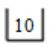→ '5' is pushed in the stack→ '+' comes so addition will be done by popping the top two elements in the stackand the result is pushed back into the stack, i.e., 10+5 = 15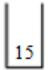→ 60 pushed in the stack→ 6 pushed in the stack→ '/' comes. So, 60/6 = 10→ '*' comes. So, 15 * 10 = 150→ '8' comes, push in the stack→ '-' comes. So, 150-8 = 142So the final result is 142.
 Question 28

Consider a CSMA/CD network that transmits data at a rate of 100 Mbps (108 bits second) over a 1 km (kilometer) cable with no repeaters. If the minimum frame size required for this network is 1250 bytes, what is the signal speed (km/sec) in the cable?

 A 8000 B 10000 C 16000 D 20000
Computer-Networks       CSMA/CD
Question 28 Explanation:
Given: L = 1250 Bytes
B = 100 Mbps
d = 1 km
v = ?
In CSMA/CD, L = 2×d/v×B
⇒ v = 2dB/L = 2×103×108/104
⇒ v = 20,000 km/sec
 Question 29

Consider a software program that is artificially seeded with 100 faults. While testing this program, 159 faults are detected, out of which 75 faults are from those artificially seeded faults. Assuming that both are and seeded faults are of same nature and have same distribution, the estimated number of undetected real fault is _____ .

 A 175 B 176 C 177 D 178
Software-Engineering       SE
Question 29 Explanation:
Note: Out of Syllabus.
 Question 30

Consider a binary tree T that has 200 leaf nodes. Then, the number of nodes in T that have exactly two children are ____.

 A 199 B 198 C 197 D 196
Data-Structures       Binary-Trees
Question 30 Explanation:
A binary tree T with n leaf nodes have exactly (n - 1) nodes that have exactly two children.
 Question 31

Let L be the language represented by the regular expression Σ*0011Σ* where Σ = {0,1}. What is the minimum number of states in a DFA that recognizes(complement of L)?

 A 4 B 5 C 6 D 8
Theory-of-Computation       Regular-Expressions
Question 31 Explanation:
No. of states in DFA accepting L and complement of L is same. So let's draw minimal DFA for L,So, 5 states are there.
 Question 32

If for non-zero x,where a≠b thenis

 A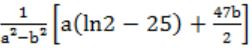BCDEngineering-Mathematics       Calculus
Question 32 Explanation:
Given,
af(x) + bf(1/x) = 1/x - 25 ------ (1)
Put x = 1/x,
af(1/x) + bf(x) = x - 25 ----- (2)
Multiply equation (1) with 'a' and Multiply equation (2) with 'b', then
abf(1/x) + a2 = a/x - 25a ----- (3)
abf(1/x) + b2 = bk - 25b ----- (4)
Subtract (3) - (4), we get
(a2 - b2) f(x) = a/x- 25a - bx + 25b
f(x) = 1/(a2 - b2) (a/x - 25a - bx +25b)
Now from equation,Hence option (A) is the answer.
 Question 33

Consider the following grammar G.

```  S → F ⎪ H
F → p ⎪ c
H → d ⎪ c```

Where S, F and H are non-terminal symbols, p, d and c are terminal symbols. Which of the following statement(s) is/are correct?

S1: LL(1) can parse all strings that are generated using grammar G.
S2: LR(1) can parse all strings that are generated using grammar
 A Only S1 B Only S2 C Both S1 and S2 D Neither S1 nor S2
Compiler-Design       Parsers
Question 33 Explanation:
For LL(1),
For first production,So, there is 'c' common in both the first(s) in the production of S. So not LL(1).
For LR(1),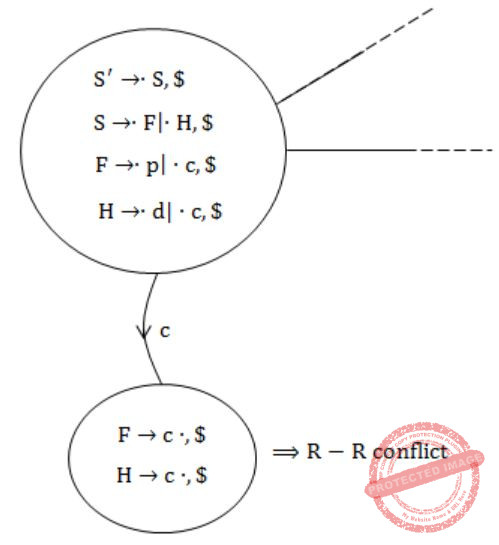Since R-R conflict is present. So, not LR(1).
Hence, Option (D) is the correct answer.
 Question 34

Consider the following partial Schedule S involving two transactions T1 and T2. Only the read and the write operations have been shown. The read operation on data item P is denoted by read(P) and the write operation on data item P is denoted by write(P).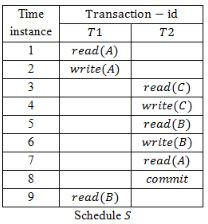A T2 must be aborted and then both T1 and T2 must be re-started to ensure transaction atomicity B Schedule S is non-recoverable and cannot ensure transaction atomicity C Only T2 must be aborted and then re-started to ensure transaction atomicity D Schedule S is recoverable and can ensure atomicity and nothing else needs to be done
Database Management System       Transactions
Question 34 Explanation:
T2 is reading the value written by T1 and getting committed before T1 commits. So it is non-recoverable schedule.
 Question 35

Consider a B+ tree in which the search key is 12 bytes long, block size is 1024 bytes, record pointer is 10 bytes long and block pointer is 8 bytes long. The maximum number of keys that can be accommodated in each non-leaf node of the tree is ____ .

 A 50 B 60 C 70 D 80
Database Management System       B+-Trees
Question 35 Explanation:
Explanation: Suppose that ‘k’ is order of the non-leaf node
k(8) + (k-1)12 ≤ 1024
20k ≤1036
k ≤ ⌊1036/20⌋ ⇒ k ≤ 51
As the order is 51, maximum we can store 50 keys.
 Question 36

Suppose Xi for i = 1, 2, 3 are independent and identically distributed random variables whose probability mass functions are Pr[Xi = 0] = Pr[Xi = 1]=1/2 for i = 1, 2, 3. Define another random variable Y = X1X2 ⊕ X3, where ⊕ denotes XOR. Then Pr[Y = 0|X3 = 0] = _______________.

 A 0.75 B 0.76 C 0.77 D 0.78
Database-Management-System       Probability
Question 36 Explanation: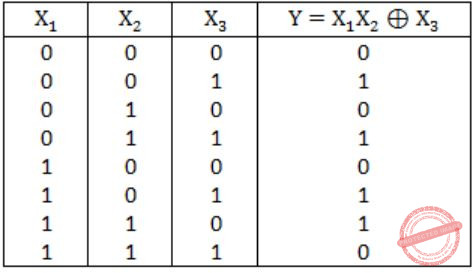Pr(Y=0/ X3=0) = Pr(Y=0 ∩ X3=0)/ Pr(X3=0)
= 3/8 / 4/8 = 3/4 = 0.75
 Question 37

The total number of prime implicants of the function f(w,x,y,z) = Σ(0, 2, 4, 5, 6, 10) is ______.

 A 3 B 4 C 2 D 1
Digital-Logic-Design       K-Map
Question 37 Explanation:Total 3 prime implicants are there.
 Question 38

Consider the following recursive C function. If get(6) function is being called in main() then how many times will the get() function be invoked before returning to the main()?

void get (int n)
```{
if (n < 1) return;
get(n-1);
get(n-3);
printf("%d", n);
}```
 A 15 B 25 C 35 D 45
Programming       Programming
Question 38 Explanation:Question 39

Assume that a mergesort algorithm in the worst case takes 30 seconds for an input of size 64. Which of the following most closely approximates the maximum input size of a problem that can be solved in 6 minutes?

 A 256 B 512 C 1024 D 2048
Algorithms       Merge-Sort
Question 39 Explanation:
Time complexity of merge sort = O(n log n) = an log n
where c is some constant
It is given that for n = 64,
cn log n = 30
c × 64 log 64 = 30
c × 64 × 6 = 30
c = 5/64
Now, the value of n for
c n log n = 360
5/64 × n log n = 360
n log n = 360×64/5
= 72 × 64 = 29 × 9
So for n = 29, it satisfies.
So, n = 29 = 512
 Question 40

The velocity v (in kilometer/minute) of a motorbike which starts from rest, is given at fixed intervals of time t(in minutes) as follows:

```t   2  4  6  8  10  12  14  16 18 20
v  10  18 25 29 32  20  11  5  2  0```

The approximate distance (in kilometers) rounded to two places of decimals covered in 20 minutes using Simpson’s 1/3rd rule is _________.

 A 309.33 B 309.34 C 309.35 D 309.36
Engineering-Mathematics       Simpson\'s-1/3rd-rule
Question 40 Explanation:
Let ‘S’ be the distance covered in 20 minutes, then by simpson’s 1/3rd rule,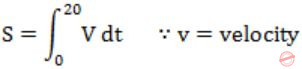∵v = velocity
= 2/3[(0+0)+4(10+25+32+11+2)+2(18+29+20+5)]
= 309.33 km
(Here length of each of the subinterval is h = 2)
 Question 41

Consider the following C program.

```# include
int main( )
{
static int a[] = {10, 20, 30, 40, 50};
static int *p[] = {a, a+3, a+4, a+1, a+2};
int **ptr = p;
ptr++;
printf("%d%d", ptr - p, **ptr};
}```

The output of the program is _________.

 A 140 B 150 C 160 D 170
Programming       Programming
Question 41 Explanation:**ptr = 40
∴ printf (“%d%d”, p + r – p, p + r) will print 140.
 Question 42

Consider the following policies for preventing deadlock in a system with mutually exclusive resources.

I. Processes should acquire all their resources at the beginning of execution. If any resource is not available, all resources acquired so far are released.
II. The resources are numbered uniquely, and processes are allowed to request for resources only in increasing resource numbers.
III. The resources are numbered uniquely, and processes are allowed to request for resources only in decreasing resource numbers.
IV. The resources are numbered uniquely. A process is allowed to request only for a resource with resource number larger than its currently held resources.

Which of the above policies can be used for preventing deadlock?

 A Any one of I and III but not II or IV B Any one of I, III, and IV but not II C Any one of II and III but not I or IV D Any one of I, II, III, and IV
Question 42 Explanation:
 Question 43

Let G be a connected undirected graph of 100 vertices and 300 edges. The weight of a minimum spanning tree of G is 500. When the weight of each edge of G is increased by five, the weight of a minimum spanning tree becomes __________.

 A 995 B 996 C 997 D 998
Algorithms       Minimum-Spanning-Tree
Question 43 Explanation:
G has 100 vertices ⇒ spanning tree contain 99 edges given, weight of a minimum spanning tree of G is 500 since, each edge of G is increased by five. ∴ Weight of a minimum spanning tree becomes 500 + 5 ⨯ 99= 995
 Question 44

If the following system has non-trivial solution,

px + qy + rz = 0
qx + ry + pz = 0
rx + py + qz = 0

then which one of the following options is True?

 A p-q+r = 0 or p = q = -r B p+q-r = 0 or p = -q = r C p+q+r = 0 or p = q = r D p-q+r = 0 or p = -q = -r
Engineering-Mathematics       Linear-Algebra
Question 44 Explanation:
For non-trivial solution the value of determinant should be zero.Question 45

Consider a network connected two systems located 8000 kilometers apart. The bandwidth of the network is 500 × 106 bits per second. The propagation speed of the media is 4 × 106 meters per second. It is needed to design a Go-Back-N sliding window protocol for this network. The average packet size is 107 bits. The network is to be used to its full capacity. Assume that processing delays at nodes are negligible. Then the minimum size in bits of the sequence number field has to be ___________.

 A 8 B 7 C 6 D 5
Computer-Networks       Sliding-Window-Protocol
Question 45 Explanation: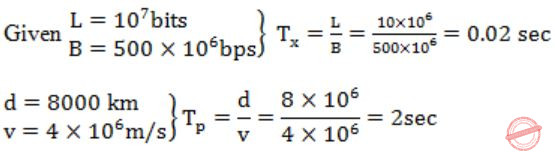η=100%
n = ?
∴a=Tp/Tn =2/0.02=100
Given Protocol, Go-back-N protocol, So η = w/(1+2a) where w = 2n-1
100/100 = w/(1+2a) ⇒ w = 1+2a
⇒ 2(n-1) = 1+2(100)
⇒ 2n - 1 = 201
⇒ 2n = 202 ⇒ 2n = 28
⇒ n = 8
 Question 46

Language L1 is polynomial time reducible to language L2. Language L3 is polynomial time reducible to L2, which in turn is polynomial time reducible to language L4. Which of the following is/are True?

I. If L4 ∈ P, L2 ∈ P
II. If L1 ∈ P or L3 ∈ P, then L2 ∈ P
III. L1 ∈ P, if and only if L3 ∈ P
IV. If L4 ∈ P, then L1 ∈ P and L3 ∈ P
 A II only B III only C I and IV only D I only
Theory-of-Computation       Reducibility
Question 46 Explanation:
L2 ≤ pL4
L1 ≤ pL2
If L4 ∈ P then L2 ∈ P hence L1 ∈ P, hence option C.
 Question 47

Consider the following reservation table for a pipeline having three stages S1, S2 and S3.

```     Time -->
-----------------------------
1    2   3    4     5
-----------------------------
S1  | X  |   |   |    |  X |
S2  |    | X |   | X  |    |
S3  |    |   | X |    |    |```

The minimum average latency (MAL) is ________.

 A 4 B 5 C 6 D 7
Computer-Organization       Pipelining
Question 47 Explanation:
Minimum average latency is based on an advanced concept in pipelining.
S1 is needed at time 1 and 5, so its forbidden latency is 5-1 = 4.
S2 is needed at time 2 and 4, so its forbidden latency is 4-2 = 2.
So, forbidden latency = (2,4,0) (0 by default is forbidden)
Allowed latency = (1,3,5) (any value more than 5 also).
Collision vector (4,3,2,1,0) = 10101 which is the initial state as well.
From initial state we can have a transition after "1" or "3" cycles and we reach new states with collision vectors
(10101 >> 1 + 10101 = 11111) and (10101 >> 3 + 10101 = 10111) respectively.
These 2 becomes states 2 and 3 respectively.
For "5" cycles we come back to state 1 itself.
From state 2 (11111), the new collision vector is 11111.
We can have a transition only when we see first 0 from right.
So, here it happens on 5th cycle only which goes to initial state. (Any transition after 5 or more cycles goes to initial state as we have 5 time slices).
From state 3 (10111), the new collision vector is 10111.
So, we can have a transition on 3, which will give (10111 >> 3 + 10101 = 10111) third state itself. For 5, we get the initial state.
Thus all the transitions are complete. State\Time 1 3 5 1 (10101) 2 3 1 2 (11111) - - 1 3 (10111) - 3 1 So, minimum length cycle is of length 3 either from 3-3 or from 1-3. So the minimum average latency is also 3.
 Question 48

In the network 200.10.11.144/27, the fourth octet (in decimal) of the last IP address of the network which can be assigned to a host is ____________.

 A 158 B 157 C 156 D 155
Computer-Networks       Subnetting
Question 48 Explanation:
No. of bit in HID part = 32-27 = 5 bits
Do AND with given IP and subnet mask then we get NID 200.10.11.128
In fourth octet first three bit will fixed for subnet and remaining 5 bits is for HID, so maximum value as 11111.
The address with all 1s in host part is broadcast address and can't be assigned to a host.
So the maximum possible last octal in a host IP is 10011110 which is 158.
 Question 49

Which of the following languages are context-free?

L1 = {ambnanbm ⎪ m, n ≥ 1}
L2 = {ambnambn ⎪ m, n ≥ 1}
L3 = {ambn ⎪ m = 2n + 1}
 A L1 and L2 only B L1 and L3 only C L2 and L3 only D L3 only
Theory-of-Computation       Context-Free-Language
Question 49 Explanation:
L1: First push all the a's in the stack then push all the b's in the stack. Now pop all the b's from the stack watching next no. of a's. And then pop all the a's from the stack watching next no. of b's. So can be done by PDA, hence CFL.
L2: First push all the a's in the stack then push all the b's in the stack. Now again a's come which cannot be compared by previous a's in the stack because at top of the stack's there are b's which is also needed to be pushed for further comparison with the next b's. So not CFL.
L3: First simply read one 'a', then push one 'a' in the stack after reading two a's and then pop all the a's by reading the b's. Since can be done by PDA hence CFL.
 Question 50

Consider the following C program:

```# include
int main( )
{
int i, j, k = 0;
j = 2 * 3 / 4 + 2.0 / 5 + 8 / 5;
k  -= --j;
for (i = 0; i < 5; i++)
{
switch(i + k)
{
case 1:
case 2: printf("n%d", i + k);
case 3: printf("n%d", i + k);
default: printf("n%d", i + k);
}
}
return 0;
}
```

The number of times printf statement is executed is __________.

 A 10 B 11 C 12 D 13
Programming       Programming
Question 50 Explanation:
j = 2*3 / 4+2.0 / 5+8 / 5;
= 6 / 4+2.0 / 5+1;
= 1 + 0.4 + 1
= 2.4
But since j is integer,
j=2
Now,
k = k - (--j)
k = 0 - (1) = -1
When i=0, i+k = -1,
printf executed 1 time
When i=1, i+k = 0,
printf executed 1 time
When i=2, i+k = 1,
printf executed 3 times
When i=3, i+k = 2,
printf executed 3 times
When i=4, i+k = 3,
printf executed 2 times
∴ Total no. of times printf executed is,
1 + 1 + 3 + 3 + 2 = 10
 Question 51

Consider the following C program. The output of the program is __________.

```# include
int f1(void);
int f2(void);
int f3(void);
int x = 10;
int main()
{
int x = 1;
x += f1() + f2() + f3() + f2();
pirntf("%d", x);
return 0;
}

int f1()
{
int x = 25;
x++;
return x;
}

int f2( )
{
static int x = 50;
x++;
return x;
}

int f3( )
{
x *= 10;
return x;
}
```
 A 230 B 240 C 250 D 260
Programming       Programming
Question 51 Explanation:
x = x + f1( ) + f2( ) + f3( ) + f4( )
f1( ) = 25 + 1 = 26
f2( ) = 50 + 1 = 51
f3( ) = 10 × 10 = 100
f2( ) = 51 × 1 = 52 (Since here x is static variable so old value retains)
∴ x = 1+26+51+100+52 = 230
 Question 52

Consider the following code sequence having five instructions I1 to I5. Each of these instructions has the following format.

```
OP Ri, Rj, Rk
```

where operation OP is performed on contents of registers Rj and Rk and the result is stored in register Ri.

```
I1 : ADD R1, R2, R3
I2 : MUL R7, R1, R3
I3 : SUB R4, R1, R5
I4 : ADD R3, R2, R4
I5 : MUL R7, R8, R9
```

Consider the following three statements:

```
S1: There is an anti-dependence between instructions I2 and I5.
S2: There is an anti-dependence between instructions I2 and I4.
S3: Within an instruction pipeline an anti-dependence always creates one or more stalls.
```

Which one of above statements is/are correct?

 A Only S1 is true B Only S2 is true C Only S1 and S3 are true D Only S2 and S3 are true
Computer-Organization       Pipelining
Question 52 Explanation:
S1: False. Antidependency means WAR dependency. There is no WAR dependency between I2 and I5.
S2: True. There is WAR dependency between I2 and I4.
S3: False. Because WAR or antidependency can be resolved by register renaming.
 Question 53

Two hosts are connected via a packet switch with 107 bits per second links. Each link has a propagation delay of 20 microseconds. The switch begins forwarding a packet 35 microseconds after it receives the same. If 10000 bits of data are to be transmitted between the two hosts using a packet size of 5000 bits, the time elapsed between the transmission of the first bit of data and the reception of the last bit of the data in microseconds is _______.

 A (1575) B (1576) C (1577) D (1578)
Packet-Switching
Question 53 Explanation:
Given that there is a single switch between the source and destination.
Bandwidth of the each link is 107 bits/sec.
Given that propagation delay between host to switch and switch to host is same i.e. 20 microseconds.
Given that 35 microseconds of buffering time is required by the switch.
Total data we need to send is 10000 bits.
Given that packet size is 5000 bits.
Number of packets we need to send is 10000/5000 = 2 packets.
Total time for the first packet to reach from source to switch = Transmission time + propagation time
Transmission time=(5000 bits)/(107 bits/sec) = 500 microseconds
Total time between source to switch is = 500 + 20 = 520 microseconds
Time required for the packet to reach from switch to destination is = Buffer time+Transmission time + propagation time = 35 + 500 + 20 = 555 microseconds.
Total time required for the first packet to reach to destination from the source = 520 + 555 =1075 microseconds.
While transferring first packet from switch to destination source starts sending of it's second packet to switch, that means from 1055 microsecond on wards transmission of 2nd packet is started by the source.
By the time first packet is reached to the destination 2nd packet is completely available at the switch.
Time required for the 2nd packet to reach to the destination from the switch is
= transmission time + propagation time = 500+20 = 520 microseconds
Therefore total time required for the two packets to reach to destination from the source = 1055+ 520 = 1575 microseconds.
 Question 54

Let R be a relation on the set of ordered pairs of positive integers such that ((p,q),(r,s)) ∈ R if and only if p - s = q - r. Which one of the following is true about R?

 A Both reflexive and symmetric B Reflexive but not symmetric C Not reflexive but symmetric D Neither reflexive nor symmetric
Engineering-Mathematics       Set-Theory
Question 54 Explanation:
Since p-q ≠ q-p
∴(p,q) R (p,q)
⇒ R is not reflexive.
Let (p,q) R (r,s) then p-s = q-r
⇒ r-q = s-p
⇒ (r,s) R (p,q)
⇒ R is symmetric.
 Question 55

For the processes listed in the following table, which of the following scheduling schemes will give the lowest average turnaround time?A First Come First Serve B Non-preemptive Shortest Job First C Shortest Remaining Time D Round Robin with Quantum value two
Operating-Systems       Process-Scheduling
Question 55 Explanation:FCFS:TAT for A = completion time(A) - AT(A) = 3 - 0 = 3
TAT of B = 9 - 1 = 8
TAT of C = 13 - 4 = 9
TAT of D = 15 - 6 = 9
∴ Avg. TAT = 29/4
SJF: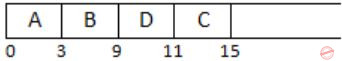TAT of A = 3 - 0 = 3
TAT of B = 9 - 1 = 8
TAT of C = 15 - 4 = 11
TAT of D = 11 - 6 = 5
∴ Avg. TAT = 27/4
SRTF:TAT of A = 3 - 0 = 3
TAT of B = 15 - 1 = 14
TAT of C = 8 - 4 = 4
TAT of D = 10 - 6 = 4
∴ Avg. TAT = 25/4
RR:TAT of A = 5 - 0 = 5
TAT of B = 15 - 1 = 14
TAT of C = 13 - 4 = 9
TAT of D = 11 - 6 = 5
∴ Avg. TAT = 33/4
∴ SRTF has lowest Avg. TAT.
There are 55 questions to complete.